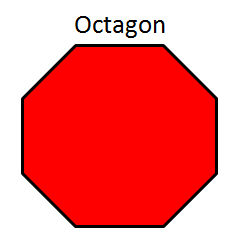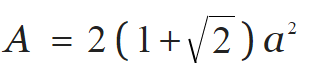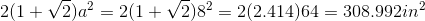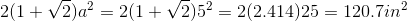# Area & Angles of an Octagon

Instructor: Nola Bridgens

Nola has taught elementary school and tutored for four years. She has a bachelor's degree in Elementary Education, a master's degree in Marketing, and is a certified teacher.

In this lesson, we will learn how to calculate the area of an octagon and measure its interior angles. We will also learn how to apply this information to solve everyday problems.

## Octagons

Kevin is creating a Lego city and wants to make a unique building in the shape of an octagon, or an 8-sided figure. He has enough pieces to make each side 8 inches long, but only has an area of 200 square inches to work in. How long should he make each side, 5 inches or 8 inches? He is also curious what the angle of each corner will be. Can you help him out?## Area of an Octagon

The formula for the area of an octagon is 2 times (1 + the square root of 2) times the length of the side squared. Here, a is the length of one side.Let's see what the area of an octagon is where each side is 8 inches.• 1 + the square root of 2 = 2.414.
• 8 squared = 64.
• 2 x 2.414 x 64 = 308.992 square inches.

As 308.992 square inches is too big for the space Kevin has to make his building, the 8-inch sides will not work. Now let's try the formula using 5 inches for each side.• 1 + the square root of 2 = 2.414.
• 5 squared = 25.
• 2 x 2.414 x 25 = 120.7 square inches.

As 120.7 square inches is less than 200 square inches, Kevin should create an octagon with 5-inch sides.

## Angles of an Octagon

The general rule for finding the interior angles, or the angles inside of a polygon, is to remember that a triangle (3-sided figure) has an angle sum of 180 degrees. Each time you add a side to a polygon, add an additional 180 degrees.

For example, since a square has one more side than a triangle, add 180 degrees to the triangle's 180 degrees. As 180 + 180 = 360, a square has interior angles of 360 degrees.

To unlock this lesson you must be a Study.com Member.

### Register to view this lesson

Are you a student or a teacher?

#### See for yourself why 30 million people use Study.com

##### Become a Study.com member and start learning now.
Back
What teachers are saying about Study.com

### Earning College Credit

Did you know… We have over 200 college courses that prepare you to earn credit by exam that is accepted by over 1,500 colleges and universities. You can test out of the first two years of college and save thousands off your degree. Anyone can earn credit-by-exam regardless of age or education level.